# Dirichlet Laplacian

(diff) ← Older revision | Latest revision (diff) | Newer revision → (diff)

Dirichlet–Laplace operator

In a broad sense, a restriction of the Laplace operator to the space of functions satisfying (in some sense) homogeneous Dirichlet boundary conditions. For an open setin, the Dirichlet Laplacian is usually defined via the Friedrichs extension procedure. Namely, first consider the (negative) Laplace operator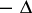defined on the subspace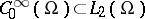of all infinitely smooth functions with compact support in. This is a symmetric operator, and the associated quadratic form (with the same domain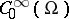) is given by the Dirichlet integral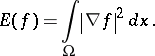(a1)

Then the formis closeable with respect to the norm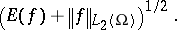The domain of its closure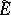is the Sobolev space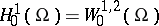. Then(given again by the right-hand side of (a1)) is the quadratic form of a non-negative self-adjoint operator (denoted by); moreover,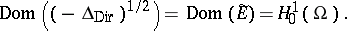The operator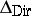(sometimes taken with the minus sign) is called the Dirichlet Laplacian (in the weak sense).

Ifis bounded domain with boundaryof class, then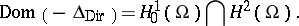The Dirichlet Laplacian for a compact Riemannian manifold with boundary is defined similarly.

For a bounded open setin,is a positive unbounded linear operator inwith a discrete spectrum (cf. also Spectrum of an operator). Its eigenvalues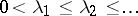(written in increasing order with account of multiplicity) can be found using the Rayleigh–Ritz variational formula (or max-min formula)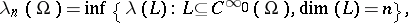where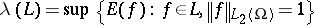for a finite-dimensional linear subspaceof. It follows from the Rayleigh–Ritz formula that the eigenvalues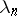are monotonically decreasing functions of. See also [a3] for a survey of the asymptotic behaviour of the eigenvalues of the Dirichlet Laplacian and operators corresponding to other boundary value problems for elliptic differential operators.

How to Cite This Entry:
Dirichlet Laplacian. Encyclopedia of Mathematics. URL: http://encyclopediaofmath.org/index.php?title=Dirichlet_Laplacian&oldid=11906
This article was adapted from an original article by M. Levitin (originator), which appeared in Encyclopedia of Mathematics - ISBN 1402006098. See original article